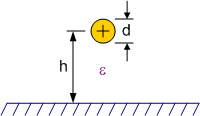# Transmission Line Equations

Transmission lines take on many forms in order to accommodate particular applications. All rely on the same basic components - two or more conductors separated by a dielectric (insulator). The physical configuration and properties of all the components determines the characteristic impedance, distortion, transmission speed, and loss.

See a discussion on transmission lines and coaxial connectors.

The following formulas are presented in a compact text format that can be copied and pasted into a spreadsheet or other application.

For the following equations, ε is the dielectric constant (ε = 1 for air)

 Two Conductors in Parallel (Unbalanced) Above Ground PlaneFor D << d, hZ0= (69/ε½) log10{(4h/d)[1+(2h/D)2]-½}Single Conductor Above Ground Plane  For d << hZ0= (138/ε½) log10(4h/d)Two Conductors in Parallel (Balanced) Above Ground PlaneFor D << d, h1, h2 Z0= (276/ε½) log10{(2D/d)[1+(D/2h)2]-½}Two Conductors in Parallel (Balanced) Different Heights Above Ground PlaneFor D << d, h1, h2Z0= (276/ε½)log10{(2D/d)[1+(D2/4h1h2)]-½}Single Conductor BetweenParallel Ground PlanesFor d/h << 0.75Z0= (138/ε½) log10(4h/πd)Two Conductors in Parallel (Balanced) Between Parallel Ground PlanesFor d << D, hZ0= (276/ε½) log10{[4h tanh(πD/2h)]/πd}Balanced Conductors BetweenParallel Ground PlanesFor d << hZ0= (276/ε½) log10(2h/πd)Two Conductors in Parallel (Balanced) of Unequal DiametersZ0= (60/ε½) cosh-1 (N)N = ½[(4D2/d1d2) - (d1/d2) - (d2/d1)]Balanced 4-Wire ArrayFor d << D1, D2Z0= (138/ε½) log10{(2D2/d)[1+(D2/D1)2]-½}Two Conductorsin Open AirZ0= 276 log10(2D/d)5-Wire ArrayFor d << DZ0= (173/ε½) log10(D/0.933d)Single Conductor inSquare Conductive EnclosureFor d << DZ0≈ [138 log10(ρ) +6.48-2.34A-0.48B-0.12C]/ε½A = (1+0.405ρ-4)/(1-0.405ρ-4) B = (1+0.163ρ-8)/(1-0.163ρ-8)C = (1+0.067ρ-12)/(1-0.067ρ-12) ρ= D/dAir Coaxial Cable withDielectric Supporting WedgeFor d << DZ0≈ [138 log10(D/d)]/[1+(ε-1)(θ/360)]½) ε = wedge dielectric constantθ= wedge angle in degreesTwo Conductors Inside Shield(sheath return)For d << D, hZ0= (69/ε½) log10[(ν/2σ2)(1-σ4)] ν = h/d       σ = h/DBalanced Shielded LineFor D>>d, h>>dZ0= (276/ε½) log10{2ν[(1-σ2)/(1+σ2)]} ν = h/d       σ = h/DTwo Conductors in Parallel (Unbalanced)Inside Rectangular EnclosureFor d << D, h, w                          ∞Z0= (276/ε½) {log10[(4h tanh(πD/2h)/πd)- ∑ log10[(1+μm2)/(1-νm2)]}                            m=1μm=sinh(πD/2h)/cosh(mπw/2h) νm=sinh(πD/2h)/sinh(mπw/2h)Equations appear in "Reference Data for Engineers," Sams Publishing 1993About RF CafeCopyright: 1996 - 2024Webmaster:    Kirt Blattenberger,    BSEE - KB3UON RF Cafe began life in 1996 as "RF Tools" in an AOL screen name web space totaling 2 MB. Its primary purpose was to provide me with ready access to commonly needed formulas and reference material while performing my work as an RF system and circuit design engineer. The World Wide Web (Internet) was largely an unknown entity at the time and bandwidth was a scarce commodity. Dial-up modems blazed along at 14.4 kbps while typing up your telephone line, and a nice lady's voice announced "You've Got Mail" when a new message arrived... All trademarks, copyrights, patents, and other rights of ownership to images and text used on the RF Cafe website are hereby acknowledged. My Hobby Website:  AirplanesAndRockets.comPlease Support RF Cafe by purchasing my  ridiculously low−priced products, all of which I created. These Are Available for Free